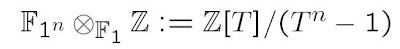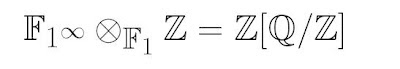## Wednesday, June 4, 2008

### F_un : day two...

By A. C. and K. Consani

On the second day of the workshop we presented the main results contained in our recent preprint (joint work with Matilde Marcolli) "Fun with F_1". The pdf file is downloadable here. Our main point is to establish a bridge between F_1 and the quantum statistical mechanical system (known as the BC-system) which gives, after passing to the dual system, a spectral realization of the zeros of the Riemann zeta function, as well as a trace formula interpretation of the Riemann-Weil explicit formulas. While a precise algebraic definition of F_1 is still elusive and premature, the section 13 of the paper "Sur les analogues algébriques des groupes semi-simples complexes" of J. Tits gives the construction of the geometries corresponding to the classical Dynkin diagrams starting from the 4 basic rank two cases: A_1 x A_1, A_2, B_2, G_2 which (over F_1) are associated to the geometries of the polygons with 2, 3, 4 and 6 sides. A striking result of J. Tits is that the groups associated to the geometries over F_1 are the Weyl groups. For instance GL_n(F_1) is the symmetric group S_n of permutations of n letters. Thus, in particular the series A_n suggests to view the category of "finite dimensional vector spaces over F_1" as the category of finite sets (with an additional "0" element). Further unpublished work of Kapranov and Smirnov suggests that, similarly to what happens for F_q, one should have for each integer n an extension F_1^n of degree n of F_1 and the category of "finite dimensional vector spaces over F_1^n" should be that of finite sets with a free action of the cyclic group Z/n of order n (still with a "0" added). This lead Soulé to the following equation :which provides a definition of the ring obtained by "extension of scalars" from F_1 to Z. Now, for q a prime power, the extensions F_q^n can be organized (after choosing an algebraic closure of F_q) as an inductive system with inclusion of F_q^n in F_q^m when n divides m. For such a pair n,m one has similarly a natural inclusion of the cyclic group Z/n in the cyclic group Z/m which relates (by two adjoint functors) the categories of "finite dimensional vector spaces" over F_1^n and F_1^m. Thus one getsBut the algebra on the right hand side is the abelian part of the BC-system which thus arises naturally by extension of scalars from the field with one element.. The inductive structure of the abelian part of the endomotive corresponds to the tower of finite extensions F_1^n, while the endomorphisms reflect the Frobenius correspondences. This gives in particular an explicit model over the integers for the BC endomotive, which is related to the original Hecke algebra description. Finally, we described the reduction at a prime of the endomotive and of the corresponding noncommutative crossed product algebra.

The talk by Henri Moscovici "Spectral triples and Q-lattices" constructs the missing type-III (twisted) spectral triple for the GL(2) analogue of the BC-system. Here is his summary:
"The two-part paper: Modular Hecke algebras and their Hopf symmetry; Rankin-Cohen brackets and the Hopf algebra of transverse geometry, (Moscow Math. J. 4 (2004), no. 1) exhibited several striking geometric features of the algebra generated by modular forms and Hecke operators. They all arose from the observation that the Hopf algebra encoding the transverse symmetries of codimension one foliations admits a natural action on this modular Hecke algebra and on its compressions.
The picture that emerged was that of a surprisingly close analogy with the transverse geometry of a codimension 1 foliation. This lecture outlines the construction of a Dirac twisted spectral triple, essentially obtained by replacing the usual Poincaré metric of the canonical bundle over the (connected component of the) Shimura variety with the Ramanujan metric defined in terms of the Dedekind eta function.
This type III spectral triple should provide the missing analytic framework for the underpinning geometry and explain its main attributes: the circle analogy, the arithmetic transgression of the Euler class, and the pseudodifferential calculus underlying the Rankin-Cohen deformations of the modular Hecke algebras."

Gunther Cornelissen's talk "Some Riemann Zeros for Function Fields" explained some computations for the space of toroidal automorphic forms. The following is his summary. "In the 1970s, Don Zagier suggested the following: a formula of Hecke proves that a GL_2 Eisenstein series of weight s integrates over a non-split torus to (almost) the zeta function of the corresponding quadratic field extension. Therefore, if we consider the space T of automorphic forms such that all these integrals vanish, and this space has a unitary structure, then the Riemann hypothesis (and simplicity of the zeros) would follow. I compute this space of so-called toroidal automorphic forms for the function field of three particular elliptic curves over finite fields. Here, the Riemann hypothesis is easily verified using the point-counting interpretation of the zeta function. In the talk, however, no point counting interpretation occured. Instead, only the Tate integral definition of zeta has been used. The condition that the toroidal integral vanishes for the constant extension torus is translated into an infinite system of linear equations in values of the automorphic form at the vertices of a Bruhat-Tits tree (for this, we need to iterate with Hecke operators). By solving this system and simultaneously the eigenvalue equation for the adjacency operator on the tree, we find that only 3 non-cusp eigenforms can occur as possible toroidal eigenforms. Since we know the eigenvalues of Eisenstein series, we can conclude that the Riemann hypothesis holds by comparing these with the possible toroidal eigenvalues.
If one does this computation for a rational function field, one can prove that its zeta function doesn't have any zeros. Now that is cracking a nut with a sledge hammer.
By some more advanced arguments (admissibility theorem of Winnie Li, integrating over other specific tori, multiplicity one for cusp forms, higher degree Hecke operators), one can even prove that in these three cases, the space of toroidal forms is one dimensional, spanned by the Eisenstein series of weight a zero of the corresponding zeta function.
References:
- paper is on arxiv:0710.2994
- thesis of Oliver Lorscheid, available from o.lorscheid@gmx.de (in this thesis, the space of toroidal forms is studied in general, and specifically computed for elliptic curves, but by a method that is a bit different, and in particular does not allow one to prove the Riemann hypothesis)."

Finally, in his talk: "Algebraic Continuation of multiple zeta values by Renormalization" Li Guo gave a remarkable application of the algebraic Birkhoff decomposition method of renormalization to the study of multiple zeta values outside the natural domain of convergence of the multiple series involved. His talk is available here.